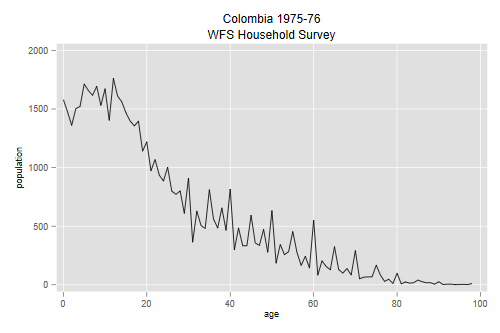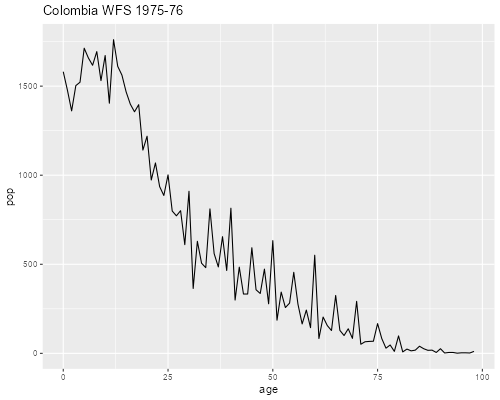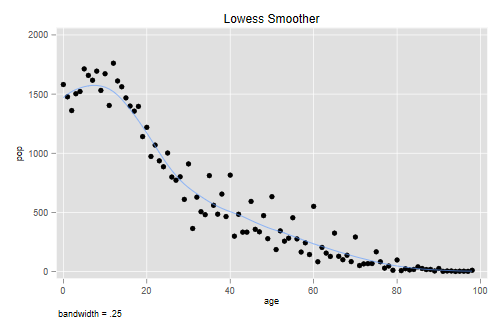Germán Rodríguez
Demographic Methods Princeton University## Smoothing: Lowess

We will work with data from the Colombia WFS Household Survey, conducted in 1975-76. I tabulated the age distribution of all household members and saved it in an ascci file, which we now read and plot:

```. infile age pop using ///
>   https://grodri.github.io/datasets/cohhpop.dat, clear

. line pop age , ///
>   title(Colombia 1975-76) subtitle(WFS Household Survey) ///
>   ytitle(population)

. graph export cohhpop.png, width(500) replace
file cohhpop.png saved as PNG format
``````> library(ggplot2)
> ggplot(co, aes(age, pop)) + geom_line() + ggtitle("Colombia WFS 1975-76")
> ggsave("cohhpopr.png", width=500/72, height=400/72, dpi=72)
```As you can see, the distribution looks somewhat less smooth than the data from the Philippines that we studied earlier. Can you compute the Myers index for this distribution?

### Running Means and Lines

The simplest way to smooth a scatterplot is to use a moving average, also known as a “running mean”. The most common approach is to use a window of 2k + 1 observations, k to the left and k to the right of each observation. The value of k is a trade off between smoothness of goodness of fit. Special care must be taken at the extremes of the range. Stata can compute running means via `lowess` with the options `mean` and `noweight`.

A common problem with running means is bias. A solution is to use weights that give more importance to the closest neighbors and less to those farther away. A popular weight function is Tukey’s tri-cube, defined as w(d) = (1-|d|3)3 for |d| < 1 and 0 otherwise, where d is the distance to the target point expressed as a fraction of the bandwidth. Stata can do this calculation via `lowess` with the option `mean` if you omit `noweight`.

An even better solution is to use running lines. We define again a neighborhood for each point, typically the k nearest neighbors on each side, fit a regression line to the points in the neighborhood, and then use it to predict a smoother value for the index observation. This sounds like a lot of work, but the calculations can be done efficiently using regression updating formulas. Stata can compute a running line via `lowess` if you omit `mean` but include `noweight`.

Better still is to use weighted running lines, giving more weight to the closest observations, which is what the lowess smoother does. A variant follows this estimation with a few iterations to obtain a more robust line. This is clearly the best technique in the family. Stata’s `lowess` uses a weighted running line if you omit `mean` and `noweight`

R implements the lowess smoother through the functions `lowess()` and the newer `loess()`, which uses a formula interface with one or more predictors and somewhat different defaults. The parameter `degree` controls the degree of the local polynomial; the default is 2 for quadratic, alternatives are 1 for linear and 0 for running means. Both implementations can use a robust estimator, with the number of iterations controlled by a parameter `iter` or `iterations`. Type `?loess` and `?lowess` in the R console for more information. In `ggplot()` you can overlay a lowess smoother by calling `geom_smooth()`.

The figure below shows the Colombian data and a lowess smoother with a span or bandwidth equal to 25% of the data.

```. lowess pop age, bwidth(0.25) gen(smooth) title(Lowess Smoother)

. graph export cohhrm.png, width(500) replace
file cohhrm.png saved as PNG format
``````> ggplot(co, aes(age, pop)) + geom_point() +
+   geom_smooth(span=0.25, se=FALSE) + ggtitle("Lowess Smoother")
> ggsave("cohhrmr.png", width=500/72, height=400/72, dpi=72)
```You may want to try different badwidths to see how the results vary.

### Digit Preference Revisited

Smoothing the age distribution provides a better way to assess digit preference than Myers’ blending. Let us compute the last digit of age and tabulate it over the entire range of the data using the observed frequencies and a lowess smoother.

```. gen lastdigit = mod(age,10)

. tab lastdigit [fw=pop], matcell(obs)

lastdigit │      Freq.     Percent        Cum.
────────────┼───────────────────────────────────
0 │      7,797       13.99       13.99
1 │      4,847        8.69       22.68
2 │      5,946       10.67       33.35
3 │      5,388        9.66       43.01
4 │      5,281        9.47       52.48
5 │      6,577       11.80       64.28
6 │      5,290        9.49       73.77
7 │      4,879        8.75       82.52
8 │      5,476        9.82       92.34
9 │      4,269        7.66      100.00
────────────┼───────────────────────────────────
Total │     55,750      100.00
```
```> library(dplyr)
> N <- sum(co\$pop)
> obs <- co |>
+   mutate(last_digit = age %% 10) |> group_by(last_digit) |>
+   summarize(prop = sum(pop)/N)
> obs
# A tibble: 10 × 2
last_digit   prop
<dbl>  <dbl>
1          0 0.140
2          1 0.0869
3          2 0.107
4          3 0.0966
5          4 0.0947
6          5 0.118
7          6 0.0949
8          7 0.0875
9          8 0.0982
10          9 0.0766
```

The raw frequencies show evidence of preference for ages ending in 0 and 5, which is very common, and probably 2 as well. We now use the smooth as weight

```. tab lastdigit [aw=smooth], matcell(fit)

lastdigit │      Freq.     Percent        Cum.
────────────┼───────────────────────────────────
0 │ 11.0366512       11.15       11.15
1 │ 10.8233452       10.93       22.08
2 │10.59322023       10.70       32.78
3 │ 10.3362373       10.44       43.22
4 │ 10.0601082       10.16       53.38
5 │ 9.77877815        9.88       63.26
6 │ 9.50162757        9.60       72.86
7 │ 9.22961745        9.32       82.18
8 │ 8.95876398        9.05       91.23
9 │ 8.68165074        8.77      100.00
────────────┼───────────────────────────────────
Total │         99      100.00
```
```> lf <- as.data.frame(lowess(co\$age, co\$pop, f = 0.25))
> M <- sum(lf\$y)
> fit <- lf |> mutate(last_digit = x %% 10) |> group_by(last_digit) |>
+   summarize(prop = sum(y)/M)
> fit
# A tibble: 10 × 2
last_digit   prop
<dbl>  <dbl>
1          0 0.114
2          1 0.111
3          2 0.108
4          3 0.105
5          4 0.101
6          5 0.0983
7          6 0.0952
8          7 0.0922
9          8 0.0894
10          9 0.0867
```

The smoothed frequencies show that we expect fewer people at higher digits, even in a smooth distribution, with more ending in 0 than 9. We are now ready to compute an index of digit preference, defined as half the sum of absolute differences between observed and smooth frequencies:

```. mata: obs = st_matrix("obs"); fit = st_matrix("fit")

. mata: sum(abs(obs/sum(obs) - fit/sum(fit)))/2
.0553043845
```
```> sum( abs(obs\$prop - fit\$prop) )/2
 0.0547919
```

We see that we would need to reshuffle 5.5% of the observations to eliminate digit preference. You may wish to compare this result with the Myers index.

